## Introduction

Fano resonances resulting from the interference between a discrete resonance and a broadband continuum of states are ubiquitous in physical science and constitute a unique resource in the field of nanophotonics1. The scattering properties of subwavelength periodic structures can be tailored by engineering their asymmetric Fano lineshapes. These extraordinary and controllable optical properties hold great promise in the context of nanophotonics, where fully integrated photonic-crystal slabs (PCS) can replace traditional Bragg multi-layers for various optical components. This approach has been successfully implemented in the context of optical filters2, switches3, sensors4, lasers5, detectors6, slow-light and non-linear devices7. It has also been proposed as a possible route to reduce thermal noise in the mirrors of large-scale gravitational-wave interferometers8.

Moreover, nanophotonics is playing an increasing role in the field of optomechanics by allowing sub-micron-scale mechanical resonators to interact via radiation pressure with highly confined optical fields. A very successful approach consists in co-localizing a photonic and a phononic mode in the small volume of a defect cavity in the plane of a PCS9, 10. In this setup, the mechanical resonator consists of a GHz internal vibrational mode localized in a very small mode volume around the defect cavity. More recently, defect-free suspended PCSs have been used as lightweight mirrors of sub-micron thickness11, 12, 13. The out-of-plane vibrational modes of the membrane can be coupled to an optical field by integrating the membrane inside a high-finesse cavity. Because of their very low mass and low mechanical frequency in the MHz range, such resonators are highly susceptible to radiation pressure. Moreover, they are good candidates to achieve hybrid optoelectromechanical transducers14, 15 because the out-of-plane vibrational modes of a suspended PCS can be simultaneously coupled with a nearby interdigitated capacitor. Such a device could have a decisive role in future (quantum) information networks because it allows up-conversion of microwave signals onto an optical carrier for subsequent detection or transmission into optical fibers.

Suspended membranes made from thin layers of high-stress materials are an advantageous platform for the development of optomechanical PCSs because they present excellent mechanical properties16. For instance, recent experiments have demonstrated Q-factors exceeding 108 with high-stress Si3N4 films at room17, 18 and cryogenic19 temperatures. Moreover, on the optical side, a stringent requirement is the resolved-sideband (or good cavity) limit20, in which the cavity storage time exceeds the mechanical period. To date, PCS optomechanical resonators have been demonstrated with one-dimensional periodic patterns (often referred to as high-contrast gratings)11, 21 or two-dimensional (2D) photonic-crystal patterns12, 13, 18 that are insensitive to the incident polarization and preserve the mechanical stiffness of the membrane. However, in these experiments, the PCS structures suffered from low optical reflectivity, precluding the operation in the resolved-sideband regime, and in most cases, the PCS was fabricated in a low stress material, preventing high mechanical quality factors.

Here we report the implementation and full optical characterization of a 2D PCS directly etched on a high-tensile stress 200 nm-thick Si3N4 nanomembrane. We reached the resolved-sideband condition for the first time with a PCS used as the end mirror of a Fabry–Perot cavity. The cavity finesse of 6390±150 is, to the best of our knowledge, an unprecedented value with free-space PCS cavities. The inferred membrane reflectivity is given by 1−R=530±25 ppm. Moreover, we measured the band diagram of the guided resonances responsible for the Fano resonances in the PCS with an angle-resolved spectrometer. In contrast with previous studies conducted in the context of PCS optical filters22 and reflectors23, we give a physical interpretation of the observed band diagram and shed new light on the physics of these structures. Finally, we introduce a theoretical description of the scattering properties of the PCS in the presence of optical losses and demonstrate that the current reflectivity is not limited by material absorption. Demonstrating high-reflectivity PCS in a high-tensile stress film, such as Si3N4, as evidenced here could also be beneficial in fields other than optomechanics, such as the generation of tunable optical filters24 or optomechanical lasers5.

## Materials and methods

### Membrane and photonic-crystal fabrication

The commercial membranes25 are made of a 200-nm stoichiometric Si3N4 film deposited on a Si substrate and released over a 1 × 1 mm square by chemical etching of the substrate (Figure 1a). The high-stress in the film ensures mechanical modes with quality factors as high as 108 at low temperature19 and ultra-low mass26. The photonic crystal is realized in the center of the membrane. It is obtained by electron-beam lithography on a layer of 200 nm of polymethyl methacrylate (PMMA) resist, followed by reactive ion etching with a plasma of CHF3 and SF6. The PMMA is then stripped off with oxygen plasma. The photonic crystal consists of a 270-μm diameter disk patterned with a square lattice of circular holes (Figure 1). The lattice parameter is Λ=830 nm, and the design value for the hole radius is 293 nm.

### PCS characterization

#### Band diagram measurement

We implemented the white-light illumination and imaging setup shown in Figure 2a to measure the PCS band diagram. A white halogen lamp is combined with a set of three lenses (L1, L2 and L3) and two diaphragms (D1 and D2) to obtain a Köhler illumination configuration. A field diaphragm (D1) conjugated to the PCS restricts the illumination to the PCS area, thus reducing the impact of light scattered on the optical elements and mounts. The aperture diaphragm D2 is used to select the incident beam angles at the PCS position. It is centered on the optical axis, and its aperture width is chosen to reject high angle-of-incidence components that are irrelevant for this experiment. A magnified image of the PCS is obtained with a microscope objective (L4) and a lens (L5). A field diaphragm (D3) sits at the image plane, conjugated to diaphragm D1, providing additional filtering of unwanted scattered light. A fiber tip is positioned in the focal plane of lens L6, that is, in the Fourier plane of this imaging system. The light collected by the fiber is guided to a near-infrared spectrometer. By scanning the fiber in the transverse (x, y) plane, the transmission spectrum can be measured at different angles. Moreover, a polarizer (Pol) is introduced between L1 and L2 for polarization selection.

#### PCS reflection, transmission and loss measurements

We implemented a single-ended cavity with the PCS as the end mirror (Figure 2b) to characterize its reflectivity, transmission and losses. The input mirror of the cavity has a 20-mm radius of curvature, and the cavity length is 17.4 mm. This ensures a waist size w0=48 μm on the PCS, smaller than the lateral extent of the photonic crystal, and an angular dispersion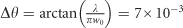rad that has a negligible influence on the optical properties of the PCS. A motorized Littman–Metcalf stabilized diode laser system from Sacher Lasertechnik GmbH (Marburg, Germany) (Lion TEC-520) is used to probe the cavity. The cavity transmission is monitored with an avalanche photodiode (Thorlabs, Newton, NJ, USA, APD110C) and is recorded continuously, while the laser wavelength is scanned over 4.8 nm. Simultaneously, the fringes of an imbalanced fiber interferometer are counted by a digital system (based on an Arduino micro-controller27) to measure the laser frequency in real time. The free-spectral range, bandwidth and visibility of each peak are extracted from Lorentzian fits.

#### Description and characterization of the PCS losses

In the following, we provide a complete theoretical description of the PCS optical properties in the presence of losses. The membrane can be described by its scattering matrix, transforming the fields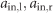incoming from left and right into outgoing fields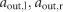(Figure 2b):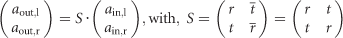where the symmetry of the membrane with respect to the xOy plane is used to assume that the direct (r,t) and reciprocal (, ) complex reflection and transmission coefficients are equal. The previous assumption allows S to be diagonalized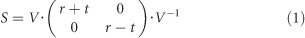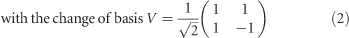For a lossless membrane, S is unitary (|r+t|=|r−t|=1), such that within a global phase factor, it is entirely determined by a single real parameter, for instance the transmission intensity T=|t|2. However, in the presence of losses, the scattered fields have a total intensity smaller than the input fields, and both eigenvalues obey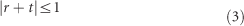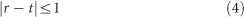Three real parameters (plus a global phase term) are required to fully describe S: in addition to the transmission intensity T, we introduce the optical losses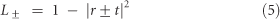associated with the symmetric and antisymmetric combinations of the left and right incoming fields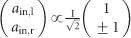. To obtain a measurement of L±, the membrane can be positioned in the center of a high-finesse cavity. This setup, initially proposed by the group of J. Harris28, consists of two single-ended cavities coupled via the transmission of the membrane. If the input and output mirrors have the same reflectivity amplitude r' and if both sub-cavities have the same length l, one obtains, in the absence of fields incident from outside the cavity (Figure 2b):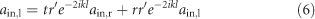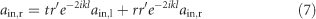where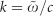is the wavenumber of the propagating field. The eigenmodes of the total optical cavity correspond to non-trivial solutions of this system for complex angular frequencies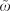. By taking the sum and difference of the previous equations, we obtain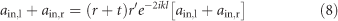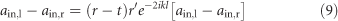Non-trivial solutions of this system correspond to symmetric (+) (antisymmetric (−)) modes with angular frequency ωp,+ (ωp,−) and damping (full width at half maximum) γ+ (γ) given by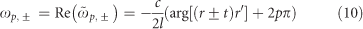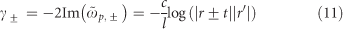where p is an integer corresponding to the longitudinal mode number. From Equation (10), the frequency splitting between the symmetric and antisymmetric modes of identical longitudinal numbers is given by: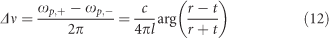Finally, if the membrane losses L± and mirror losses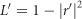are small, Equation (11) simplifies to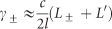Hence, the damping of the symmetric and antisymmetric modes gives direct access to the two parameters L+ and L.

To perform this measurement, a 32-mm long cavity is formed by two mirrors with transmission-limited losses L′=455 ppm and radii of curvature 20 mm. The PCS is set in the center of the cavity on a three-axis translation stage. To align the cavity axis with respect to the PCS, the end mirror is also mounted on a two-axis translation stage. Finally, the PCS frame is glued on a piezoelectric stack to allow automatic scans of its z position over several free spectral ranges.

## Results and discussion

### Band diagram of the PCS

The physics of high-contrast gratings has previously been described in detail29, 30. Here we extend it to the case of a PCS, following earlier work13, 31. The high-reflection properties of the PCS result from Fano resonances arising from an interference between the direct reflection from the PCS and light leaking out from internal high-Q guided modes in the PCS. To unveil the band structure of the guided modes, we calculate the transmission of the PCS by rigorous coupled wave analysis (RCWA)32, which is suitable for periodic structures, such as our square lattice of holes (lattice parameter Λ=830 nm, membrane thickness h=213 nm and hole radius rh=276 nm). We begin the analysis with the unpatterned membrane limit, for which the dielectric constant of both the membrane material and the holes is set to an average value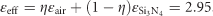The field solutions are guided modes, referred to as ‘unperturbed modes’ in the following discussion. They are plane waves reflected by total internal reflection upon the two faces of the membrane. In this regime, the unperturbed modes are evanescent outside of the membrane: the z component of their wavevector fulfils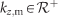inside the membrane and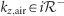in the surrounding medium. TE (TM) modes have their electric (magnetic) field within the plane of the membrane and are represented in Figure 3 in red (blue). The eight modes are sorted according to their symmetry class with respect to the xOz plane. Symmetric modes (TM(±1, 0)) are not excited under s-illumination, whereas antisymmetric modes (TE(±1, 0)) are not excited under p-illumination. The complex coefficients describing the reflection inside the membrane for the electric field are given by Fresnel’s law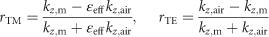We have numerically solved the round-trip resonance condition within the membrane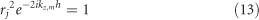for j=TE or TM and for the lowest possible value of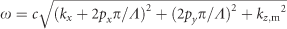, (where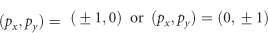are the diffraction orders in the x and y directions, respectively). Note that the periodicity Λ is not a property of the unpatterned membrane and is arbitrarily chosen to match that of the patterned membrane. Given the phases of rTE and rTM, we find that TE modes have a lower fundamental resonance frequency than TM modes. Finally, the symmetry of the mode with respect to the xOy plane is given by the sign of the half round-trip propagation coefficient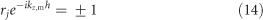From the expressions of rTE and rTM, it follows that the fundamental TE (TM) mode is antisymmetric (symmetric) with respect to xOy. The calculated mode frequencies as a function of transverse wavevector kx are represented by the lines in Figure 3c and 3d. To first order, the frequency of the modes diffracted along the y direction is not affected by the transverse kx component, contrary to the modes diffracted along the x direction.

The results of the RCWA for the patterned membrane are superimposed as a color plot in Figure 3c and 3d (the refractive index of Si3N4 is given by33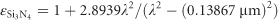. In this case, the modes described in the unpatterned case are now coupled by diffraction, giving rise to avoided crossings. In addition, the reflections at the membrane/air interfaces couple the guided modes to the zeroth-order modes propagating in free-space, leading to an effective loss channel. The guided resonances acquire a finite lifetime inversely proportional to the width of the Fano resonance (cut along a fixed value of kx in Figure 3). The lifetime of the TM modes is ~20 times that of the TE modes. At normal incidence, the yOz plane constitutes an additional plane of symmetry (antisymmetry) in the s- (p-) polarization, and, hence, only the combinations TE(1, 0)+TE(−1, 0) and TM (0,1)+TM(0, −1) (respective TE(0, 1)+TE (0, −1) and TM(1, 0)+TM(−1, 0)) are effectively coupled to free-space modes: the linewidth of the other Fano resonances vanishes close to kx=0. The white-light angle-resolved spectrometer described previously allowed us to measure the band structure of the guided modes for small transverse wavevectors kx. The experimental transmission with s- (p-) illumination is presented in Figure 3e and 3f, together with three different cuts with incidence angles 3.57°, 1.23° and 0.33°. The simulation is fitted to the data with the hole radius and membrane thickness as free parameters. The former is only specified to within 10% accuracy, and the latter may differ from the design value due to imperfect pattern transfer on electron-beam lithography and etching. The fitted values rh=276 nm and h=213 nm are in good agreement with the design values. The theoretical fits are represented in blue in Figure 3g and 3h. The reduced visibility of the peaks, particularly pronounced with the TM modes, is accounted for by convolving the theoretical map with the 4-nm wide response of the spectrometer. In the next section, we analyze in greater detail the TE Fano resonance responsible for the largest dip in transmission close to v=c × 0.929 μm−1=c/(1.076 μm) in Figure 3g and 3h.

### Detailed characterization of a Fano resonance

For optical frequencies v=c/λ close to a guided mode resonance, the PCS complex reflection and transmission coefficients r and t are given in good approximation by the simple two-mode model31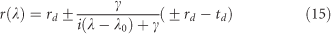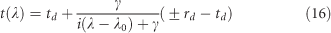where rd and td are the off-resonant coefficients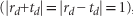, λ0 is the wavelength of the guided mode resonance, γ is the resonance width and the plus/minus sign in Equation (15) reflects the symmetry of the guided mode: as discussed in the previous section, the lowest-order TE mode is antisymmetric with respect to the xOy plane; thus, the minus sign applies. In the absence of optical losses, a striking feature of this Fano lineshape is the existence of a frequency λ1=λ0+iγrd/td for which the PCS transmission vanishes:, and, accordingly, the reflectivity tends to unity: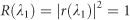.

By fitting the RCWA results with Equations (15) and (16) around the largest TE Fano resonance, we determine λ1=1076 nm and γ=12 nm. To study the optical behavior of the PCS around λ1, a single-ended optical cavity of length l0 is achieved, using the PCS as the end mirror (Figure 2). The transmission of the cavity is monitored, whereas a tunable diode laser is swept across the Fano resonance. The recorded signal, shown in Figure 4a, displays a comb of peaks spaced by the free-spectral range of the cavity given by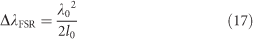The linewidth Δλn(λ) of each longitudinal cavity mode n is then obtained by fitting a Lorentzian profile to each of the individual peaks. To minimize the impact of scan-speed fluctuations, we generate 50 MHz sidebands in the laser spectrum with an electro-optic modulator for frequency calibration of the instantaneous scanning speed. Denoting the finesse of the cavity by F(λ), the total round-trip loss of the cavity is given by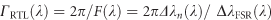. The results are plotted in blue in Figure 4b as a function of the laser wavelength. The lowest level of ΓRTL=(985±25) ppm, corresponding to a finesse of 6385±25 was found at λ1=1070.9 nm. The 5.1 nm discrepancy with respect to the results of Figure 3 is quite small considering that two different membranes from the same microfabrication run were used in these experiments. In the simulations, it is accounted for by changing the hole radius from rh=276 nm to rh=285 nm. Taking into account the 455 ppm transmission of the input mirror, we infer a membrane reflectivity as good as 1−R(λ1)=530 ppm.

To discriminate between the contributions of the membrane transmission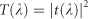and the optical losses L(λ)=1−R(λ)−T(λ) in ΓRTL(λ), we use the extra information embedded in the height of the transmitted peaks. The transmission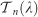of the cavity at resonance with the nth mode is given by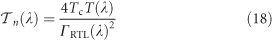where Tc is the transmission of the input mirror, assumed to be constant over the wavelength range scanned here. In the experiment, a signal proportional to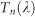is measured, so that we can deduce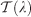from Equation (18) within a multiplicative constant α. By assuming that the losses L(λ) are within the interval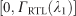at the highest accessible wavelength, we obtain a confidence interval for α and hence for T(λ)=ΓRTL(λ)−L(λ) (in green in Figure 4c and 4d) and L(λ) (in red) over the whole wavelength range. For comparison, the transmission calculated by RCWA is displayed by a green solid line in Figure 4c and 4d. The agreement between RCWA and the experimental results is excellent. At the Fano resonance λ1=1070.9 nm, the round-trip damping is vastly dominated by membrane losses. The lowest cavity bandwidth κ/2=c/4l0F(λ1)=675 kHz measured in this single-ended cavity setup approaches the resolved-sideband condition for the first-order flexure modes of the suspended membrane.

To understand the exact form of the scattering matrix and to gain insight into the origin of the losses observed in the previous experiment, a PCS is positioned in the middle of a high-finesse cavity. The PCS position Δl is scanned along the cavity axis with a piezoelectric actuator. In this configuration, the PCS couples two cavities of length l1l/2 and l2−Δl/2, where l1l2l are the initial lengths of the first and second sub-cavities, which are assumed to be simultaneously resonant with the central laser wavelength λc for Δl=0. The cavity transmission, recorded as a function of Δl and laser detuning Δv λcλ, is represented in the color plots of Figure 5a–5c. The avoided crossing of the symmetric and antisymmetric modes is visible in the center of the figure. From a Lorentzian fit of the peaks at the avoided crossing and along the vertical axis, we extract the frequency spacing Δv, and the linewidth γ± of the symmetric and antisymmetric modes. The experiment is then reproduced for several laser wavelengths λc close to the Fano resonance, and the corresponding frequency spacings and linewidths are shown in Figure 5d and 5e, respectively.

In Figure 5a and 5c, the upper and lower branches of the avoided crossing are strongly asymmetric. This is a direct consequence of the difference between L+ and L. For λc<λ1 (Figure 5a), the phase of (rt)/(r+t) is positive, such that from Equation (12), the symmetric mode (angular frequency ω+) constitutes the upper branch of the crossing, whereas the antisymmetric mode (angular frequency ω) is the lower branch. On the other hand, for λc>λ1 (Figure 5c), Δv=(ω+ω)/2π changes its sign and the upper and lower branches are exchanged. Finally, at the Fano resonance λc=λ1, r and t are in phase, so the symmetric and antisymmetric modes are perfectly degenerate. Combined with the inequalities of Equation (3), an upper bound for the transmission of the membrane can be derived: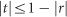at the Fano resonance. For 1−R=985 ppm, this corresponds to a transmission as low as T≤0.2 ppm. Hence, the residual transmission of 100 ppm observed in Figure 4 is in contradiction with the two-port description developed in this work. A first explanation is the possible scattering into non-overlapping spatial modes due to surface roughness. Moreover, given our experimental parameters, we expect from RCWA that the wavevector components of the input Gaussian beam not aligned with a symmetry plane of the membrane should give rise to ≈20 ppm transmission into the polarization orthogonal to the input beam (with a spatial distribution that is antisymmetric with respect to the xOz and yOz planes). Nevertheless, as shown in Figure 4, the residual transmission only accounts for a small fraction of the total cavity losses close to the Fano resonance, and the nature of these losses remains to be elucidated.

The difference between the losses L± experienced by the symmetric and antisymmetric modes is captured by a simple phenomenological model; because the Fano resonance results from the interference between direct PCS transmission and a high-Q guided resonance, it can be safely assumed that the losses mostly affect the former. Hence, Equations (15) and (16) are modified to include a phenomenological loss rate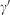in the high-Q response as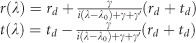Using this model, it follows that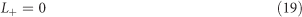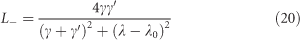thus, only the antisymmetric mode of the two-cavity setup is affected by the optical losses, with a value proportional to the Lorentzian response of the guided resonance. This is an intuitive result because the antisymmetric guided resonance under study is not driven by a symmetric incoming field (and vice versa for the symmetric TM resonance). To quantitatively evaluate the model, Figure 5e shows the total expected losses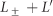, where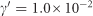nm is the only adjustable parameter because λ1=1079.1 nm, γ=12 nm and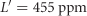are independently determined. Therefore, optical losses are found to account for <1‰ of the total decay rate of the guided mode resonance. The previous model shows that the asymmetry between L+ and L is a direct consequence of the physics of Fano resonances and is not specific to a particular loss mechanism, such as absorption or scattering, because both would preferentially affect the high-Q guided mode. This finding is in stark contrast with a model recently proposed in Ref. 21, where such an asymmetry was attributed to absorption in the material. Moreover, we show in the following that absorption should have a negligible influence on the optical loss of our PCS. If the losses observed in our experiment were entirely due to optical absorption, the value Im(n)=(3.5±1.5) × 10−5 could be derived for the absorption of the Si3N4 layer. The values of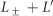obtained by RCWA simulation, including an absorption of 2 × 10−5≤Im(n)≤5 × 10−5 are located within the shaded areas in Figure 5e. Because previous experiments34 have found values for the absorption of a similar layer that are an order of magnitude smaller (Im(n)=2.0 × 10−6), we conclude that scattering is the main source of losses. In the single-ended cavity case, where the field is essentially incident on one side of the membrane, the relevant optical losses are given by L=(L++L)/2. Thus, the decrease of optical losses with optical wavelength visible in Figure 4b is consistent with Equations (19) and (20). With the previous smaller value of Si3N4 absorption, RCWA simulation shows that a reflectivity as high as R≈1–80 ppm, corresponding to a finesse close to 80 000, could be achieved by improving the PCS fabrication process.

## Conclusions

High-stress Si3N4 films have been successfully patterned to achieve a 2D PCS and have provided a detailed study of the rich mode structure underlying the high reflectivity of the crystal. We have fully characterized the scattering and loss properties of the device. We have also experimentally demonstrated the wide tunability of the membrane reflectivity via adjustment of the laser wavelength close to a Fano resonance. Such tunability is highly desirable in the context of membrane-in-the-middle optomechanics experiments. In particular, the ability to reach a very narrow energy gap between the eigenmodes of the coupled cavities can be used to enter the regime where the photon exchange time becomes comparable to the mechanical period. This would enable the observation of mechanically induced coherent photon dynamics, such as Autler–Townes splitting or Landau–Zener–Stueckelberg dynamics35. Finally, the bandwidth of the single-ended cavity described in this work, as low as 675 kHz, is in the resolved sideband regime for the first-order harmonics of the drum mode, ranging from 596 kHz for the (0, 1) mode to 1.2 MHz for the (2, 2) mode used in previous quantum optomechanics experiments16. Hence, this technique results in devices suitable for the development of optomechanical transducers.